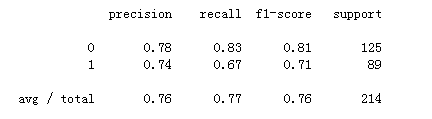Python 的sklearn库包含许多可用于机器学习的工具，本文以经典的泰坦尼克号问题为例，来说明在Python中使用sklearn做机器学习的一般流程。由于本人对机器学习了解还不深，对于本文内容如有任何建议或意见，欢迎提出！闲话少说，咱们立即进入主题。

### 1. 读取并查看数据

``` # 导入后续需要用到的库文件
import numpy as np
import pandas as pd
import seaborn as sns
import matplotlib.pylab as plt
from sklearn.preprocessing import StandardScaler
from sklearn.cross_validation import train_test_split
from sklearn.linear_model import LogisticRegression
from sklearn.metrics import confusion_matrix
from sklearn.metrics import classification_report ```

```# 读取数据并查看

```# 查看数据行列
data.shape```

```# 检查NA值的情况
data.isnull().sum()```

### 2. 特征选取

```# 选取数据集中有用的特征
data = data.drop(labels=['PassengerId', 'Name', 'Ticket', 'Cabin'], axis=1)

### 3. 缺失值处理

```# 去除有缺失值的行
data = data.dropna()```

### 4. 离散特征处理

sklearn中模型最后训练的数据需要是数值型的，因此Sex和Embarked这两列的数值需要转换为数值型。

```# 分类变量编码
data_dummy = pd.get_dummies(data[['Sex', 'Embarked']])```

# 编码后和原来其他列数据拼接

```data_conti = pd.DataFrame(data, columns=['Survived', 'Pclass', 'Age', 'SibSp', 'Parch', 'Fare'], index=data.index)
data = data_conti.join(data_dummy)```

### 5. 分割训练集和测试集

```X = data.iloc[:, 1:]
y = data.iloc[:, 0]
X_train, X_test, y_train, y_test = train_test_split(X, y, test_size=0.3, random_state=0)```

### 6. 连续特征处理

```# 标准化
stdsc = StandardScaler()
X_train_conti_std = stdsc.fit_transform(X_train[['Age', 'SibSp', 'Parch', 'Fare']])
X_test_conti_std = stdsc.fit_transform(X_test[['Age', 'SibSp', 'Parch', 'Fare']])
# 将ndarray转为dataframe
X_train_conti_std = pd.DataFrame(data=X_train_conti_std, columns=['Age', 'SibSp', 'Parch', 'Fare'], index=X_train.index)
X_test_conti_std = pd.DataFrame(data=X_test_conti_std, columns=['Age', 'SibSp', 'Parch', 'Fare'], index=X_test.index)```

```# 有序分类变量Pclass
X_train_cat = X_train[['Pclass']]
X_test_cat = X_test[['Pclass']]
# 无序已编码的分类变量
X_train_dummy = X_train[['Sex_female', 'Sex_male', 'Embarked_C', 'Embarked_Q', 'Embarked_S']]
X_test_dummy = X_test[['Sex_female', 'Sex_male', 'Embarked_C', 'Embarked_Q', 'Embarked_S']]
# 拼接为dataframe
X_train_set = [X_train_cat, X_train_conti_std, X_train_dummy]
X_test_set = [X_test_cat, X_test_conti_std, X_test_dummy]
X_train = pd.concat(X_train_set, axis=1)
X_test = pd.concat(X_test_set, axis=1)```

### 7. 建模和预测

```# 基于训练集使用逻辑回归建模
classifier = LogisticRegression(random_state=0)
classifier.fit(X_train, y_train)

# 将模型应用于测试集并查看混淆矩阵
y_pred = classifier.predict(X_test)
confusion_matrix = confusion_matrix(y_test, y_pred)
print(confusion_matrix)```

```# 在测试集上的准确率
print('Accuracy of logistic regression classifier on test set: {:.2f}'.format(classifier.score(X_test, y_test)))```

Accuracy of logistic regression classifier on test set: 0.77

`print(classification_report(y_test, y_pred))`